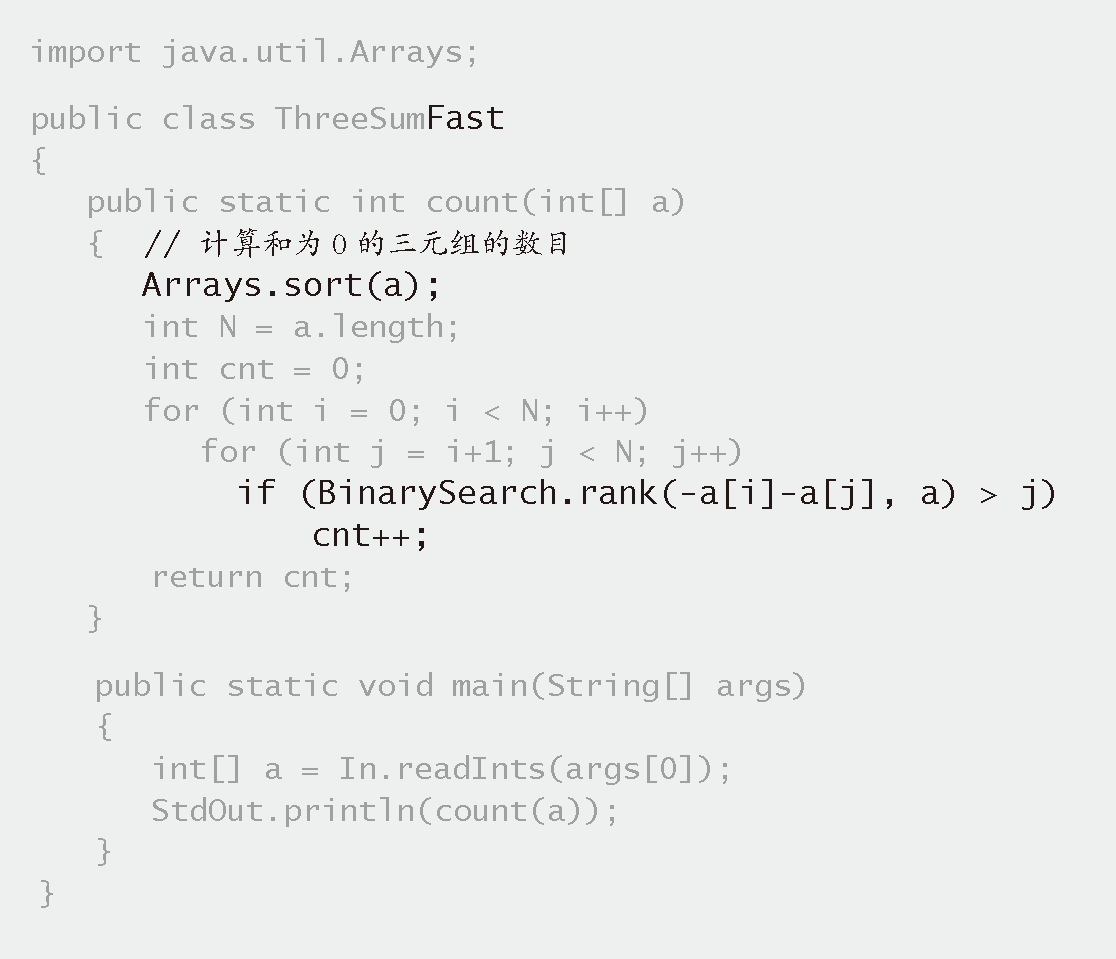# 算法（4th ed）(169)：基础——算法分析 6.5.3(设计更快的算法：下界）3-sum 问题的 $N^2\lg N$ 解法

TwoSum $N^2$
TwoSumFast $N\log N$
ThreeSum $N^3$
ThreeSumFast$N^2\log N$

• 实现并分析该问题的一种简单的解法。我们通常将它们称为暴力算法，例如 ThreeSum 和 TwoSum。
• 考查算法的各种改进，它们通常都能降低算法所需的运行时间的增长数量级，例如 TwoSumFast 和 ThreeSumFast。
• 用实验证明新的算法更快。